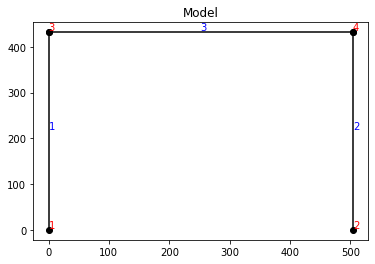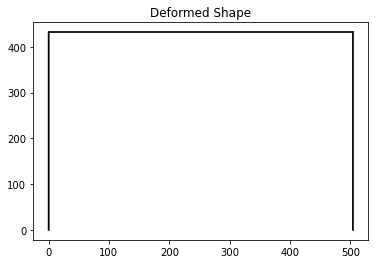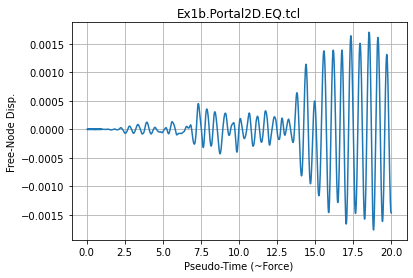# OpenSees Examples Manual Examples for OpenSeesPy

## OpenSees Example 1b. Elastic Portal Frame -- Earthquake Ground Motion

You can find the original Examples:
https://opensees.berkeley.edu/wiki/index.php/Examples_Manual
Original Examples by By Silvia Mazzoni & Frank McKenna, 2006, in Tcl
Converted to OpenSeesPy by SilviaMazzoni, 2020

## Simulation Process

Each example script does the following:

### A. Build the model

1. model dimensions and degrees-of-freedom
2. nodal coordinates
3. nodal constraints -- boundary conditions
4. nodal masses
5. elements and element connectivity
6. recorders for output

### B. Define & apply gravity load

2. static-analysis parameters (tolerances & load increments)
3. analyze
5. reset time to zero

### C. Define and apply lateral load

• Time Series and Load Pattern (nodal loads for static analysis, support ground motion for earthquake)
• lateral-analysis parameters (tolerances and displacement/time increments)

• define the displacement increments and displacement path

• define the input motion and all associated parameters, such as scaling and input type
• define analysis duration and time increment
• define damping
• analyze
• Introductory Examples The objective of Example 1a and Example 1b is to give an overview of input-file format in OpenSees using simple scripts. These scripts do not take advantage of the Tcl scripting capabilities shown in the later examples. However, they do provide starting a place where the input file is similar to that of more familiar Finite-Element Analysis software. Subsequent examples should be used as the basis for user input files.

# OpenSees Example 1b. 2D Elastic Portal Frame -- Earthquake Ground Motion

<b> Objectives of Example 1b </b>
- Two element types <br>In :
############################################################
#  EXAMPLE:
#       pyEx1b.Portal2D.EQ.tcl.py
#          for OpenSeesPy
#  --------------------------------------------------------#
#  by: Silvia Mazzoni, 2020
#       [email protected]
############################################################

# configure Python workspace
import openseespy.opensees as ops
import eSEESminiPy
import os
import math
import numpy as numpy
import matplotlib.pyplot as plt
ops.wipe()
# --------------------------------------------------------------------------------------------------
# Example 1. portal frame in 2D
# dynamic earthquake analysis of Portal Frame, with gravity.
# all units are in kip, inch, second
# elasticBeamColumn ELEMENT
# Silvia Mazzoni and Frank McKenna, 2006
#
# ^Y
# or
# 3_________(3)________4 __
# or   |  |
# or   |  |
# or   |  |
# (1)   (2) LCol
# or   |  |
# or   |  |
# or   |  |
# =1=   =2= _or_ -------->X
# or----------LBeam------------|
#

# SET UP ----------------------------------------------------------------------------
ops.wipe()     #  clear opensees model
ops.model('basic','-ndm',2,'-ndf',3)     #  2 dimensions, 3 dof per node
if not os.path.exists('Data'):
os.mkdir('Data')

# define GEOMETRY -------------------------------------------------------------
# nodal coordinates:
ops.node(1,0,0)     #  node , X Y
ops.node(2,504,0)
ops.node(3,0,432)
ops.node(4,504,432)

# Single point constraints -- Boundary Conditions
ops.fix(1,1,1,1)     #  node DX DY RZ
ops.fix(2,1,1,1)     #  node DX DY RZ
ops.fix(3,0,0,0)
ops.fix(4,0,0,0)

# nodal masses:
ops.mass(3,5.18,0.,0.)     #  node , Mx My Mz, Mass=Weight/g.
ops.mass(4,5.18,0.,0.)

# Define ELEMENTS -------------------------------------------------------------
# define geometric transformation: performs a linear geometric transformation of beam stiffness and resisting force from the basic system to the global-coordinate system
ops.geomTransf('Linear',1)     #  associate a tag to transformation

# connectivity: (make A very large, 10e6 times its actual value)
ops.element('elasticBeamColumn',1,1,3,3600000000,4227,1080000,1)     #  element elasticBeamColumn eleTag iNode jNode A E Iz transfTag
ops.element('elasticBeamColumn',2,2,4,3600000000,4227,1080000,1)
ops.element('elasticBeamColumn',3,3,4,5760000000,4227,4423680,1)

# Define RECORDERS -------------------------------------------------------------
ops.recorder('Node','-file','Data/DFreeEx1bEQ.out','-time','-node',3,4,'-dof',1,2,3,'disp')     #  displacements of free nodes
ops.recorder('Node','-file','Data/DBaseEx1bEQ.out','-time','-node',1,2,'-dof',1,2,3,'disp')     #  displacements of support nodes
ops.recorder('Node','-file','Data/RBaseEx1bEQ.out','-time','-node',1,2,'-dof',1,2,3,'reaction')     #  support reaction
ops.recorder('Element','-file','Data/FColEx1bEQ.out','-time','-ele',1,2,'globalForce')     #  element forces -- column
ops.recorder('Element','-file','Data/FBeamEx1bEQ.out','-time','-ele',3,'globalForce')     #  element forces -- beam

# define GRAVITY -------------------------------------------------------------
ops.timeSeries('Linear',1)     # timeSeries Linear 1;
ops.pattern('Plain',1,1) #
ops.eleLoad('-ele',3,'-type','-beamUniform',-7.94)     #  distributed superstructure-weight on beam

ops.wipeAnalysis()     # adding this to clear Analysis module
ops.constraints('Plain')     #  how it handles boundary conditions
ops.numberer('Plain')     #  renumber dofs to minimize band-width (optimization), if you want to
ops.system('BandGeneral')     #  how to store and solve the system of equations in the analysis
ops.test('NormDispIncr',1.0e-8,6)     #  determine if convergence has been achieved at the end of an iteration step
ops.algorithm('Newton')     #  use Newtons solution algorithm: updates tangent stiffness at every iteration
ops.integrator('LoadControl',0.1)     #  determine the next time step for an analysis,   apply gravity in 10 steps
ops.analysis('Static')     #  define type of analysis static or transient
ops.analyze(10)     #  perform gravity analysis
ops.loadConst('-time',0.0)     #  hold gravity constant and restart time

# DYNAMIC ground-motion analysis -------------------------------------------------------------
accelSeries  = 900
ops.timeSeries('Path',accelSeries,'-dt',0.01,'-filePath','BM68elc.acc','-factor',1)     #  define acceleration vector from file (dt=0.01 is associated with the input file gm)
ops.pattern('UniformExcitation',2,1,'-accel',accelSeries)     #  define where and how (pattern tag, dof) acceleration is applied
ops.rayleigh(0.,0.,0.,2*0.02/math.pow(ops.eigen('-fullGenLapack',1),0.5))     #  set damping based on first eigen mode

# create the analysis
ops.wipeAnalysis()     #  clear previously-define analysis parameters
ops.wipeAnalysis()     # adding this to clear Analysis module
ops.constraints('Plain')     #  how it handles boundary conditions
ops.numberer('Plain')     #  renumber dofs to minimize band-width (optimization), if you want to
ops.system('BandGeneral')     #  how to store and solve the system of equations in the analysis
ops.test('NormDispIncr',1.0e-8,10)     #  determine if convergence has been achieved at the end of an iteration step
ops.algorithm('Newton')     #  use Newtons solution algorithm: updates tangent stiffness at every iteration
ops.integrator('Newmark',0.5,0.25)     #  determine the next time step for an analysis
ops.analysis('Transient')     #  define type of analysis: time-dependent
ops.analyze(1000,0.02)     #  apply 1000 0.02-sec time steps in analysis

print('Done!')

Done!

In :
eSEESminiPy.drawModel()In :
# plot deformed shape at end of analysis (it may have returned to rest)
# amplify the deformtions by 5
eSEESminiPy.drawDeformedShape(5)In :
ops.wipe() # the wipe command here closes all recorder files
plt.close('all')
fname3 = 'Data/DFreeEx1bEQ.out'

End of Run: pyEx1b.Portal2D.EQ.tcl.py